EngliSea > M > Math is Fun

#math
20615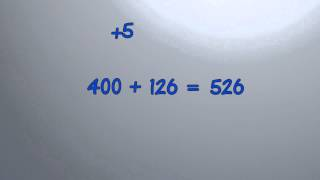addition tips and tricks 2
compensation and doubling
5048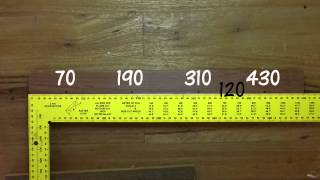arithmetic sequence
An arithmetic sequence is made by adding the same value each time. The value added each time is the common difference.
6266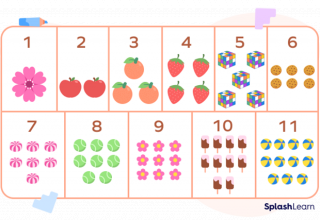○○○ cardinal number ⪢⪢
A _____ says how many of something there are. ￫ nominal, ordinal #math
7070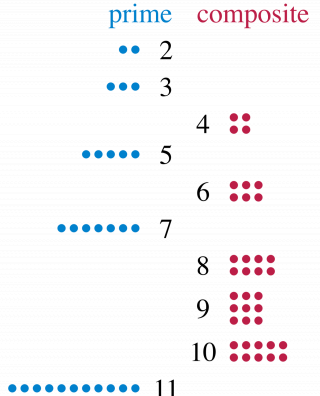○○○ composite number ⪢⪢
It is a _____ when it can be divided evenly by numbers other than 1 or itself. #math
9580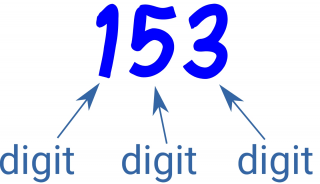◎◎◎ digit ⪢⪢
A _____ is a single symbol used to make numerals. #math
20673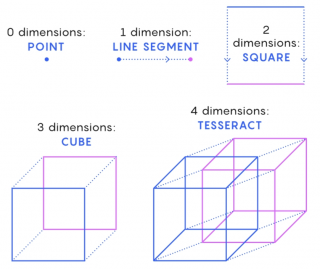◎◎◎ dimension ⪢⪢
The number of _____s is how many values are needed to locate points on a shape. #math
10324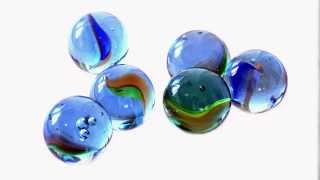◌◌◌ dimension
The number of dimensions is how many values are needed to locate points on a shape.
5044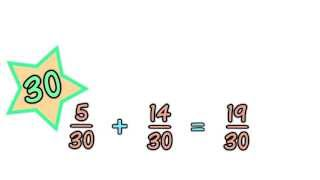least common denominator
The denominator is the bottom number in a fraction. It shows how many equal parts the item is divided into.
8951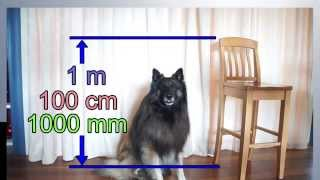metric length
We can measure how long things are, or how tall or how far apart they are. The meter is the basic measurement of length in the metric system. How far is it across this patch of grass?
5046○○○ nominal number ⪢⪢
A _____ is a number used like a name, not as an actual value or position. ￫ cardinal, ordinal #math
10307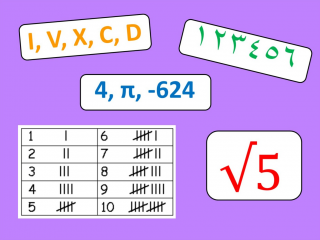◎◎◎ number ⪢⪢
A _____ is a count or measurement. It is really an idea in our minds. #math
20669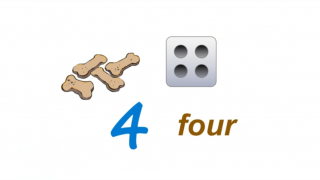○○○ number ⪢⪢
Here are some ways to show the number 4. We can even tap the ground 4 times. #math
20670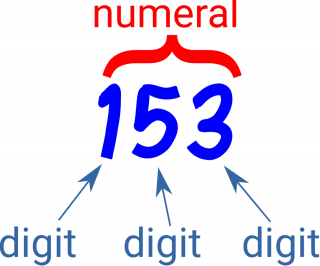◎◎◎ number ⪢⪢
Digits make up numerals and numerals stand for an idea of a _____ just like letters make up words and words stand for ideas. #math
9880○○○ numeral ⪢⪢
A _____ is a symbol or name that stands for a number. #math
20672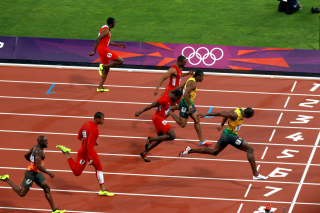○○○ ordinal number ⪢⪢
￫ cardinal, nominal #math
9858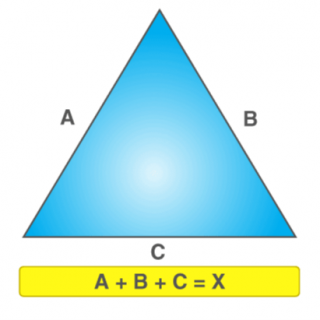○○○ perimeter ⪢⪢
The perimeter is the distance around the edge of _____ triangle. #math
18161○○○ prime number ⪢⪢
It is a _____ when it can't be divided evenly by any number except 1 or itself. #math
9581

○○○ put into practice ⪢⪢
#math
20616

○○○ put together ⪢⪢
#math
20674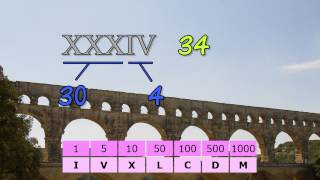Roman numeral
The Romans showed numbers into the thousands using these 7 symbols: I for 1, V for 5, X for 10, L for 50, C for 100, D for 500 and M for 1000.
5045

○○○ skip ⪢⪢
#math
20614

○○○ swap ⪢⪢
#math
20613

○○○ upwards ⪢⪢
#math
20612-